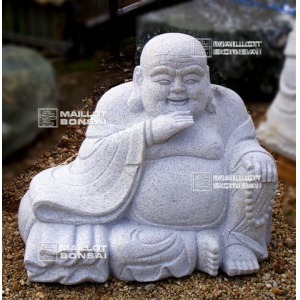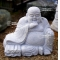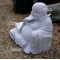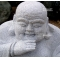##### The Japanese Bonsai specialist
Direct order Contact Help / Services Newsletter# Garden buddha statue in granite 60 cm

Statue en granitref. : 9747

936,00

voluminous and/or heavy product extra shipping of 35,00

Available quantity : 1Order

###### Description

Height 60 cm. Width: +-70 cm, depth +-40 cm. Weight 250 kilograms. This solid granite Buddha statue will transform any outdoor space into a Zen meditation garden or peaceful sanctuary. He is seated in full lotus position and his right hand is extended towards the ground, referring to when the Buddha asked mother earth to witness his enlightenment. The face has a serene expression, framed by long earlobes, and robes hang over his left shoulder. The sculptor’s tool marks are visible on the hand-carved piece (unlike sculptures made for the mass-market).

#buddha 4.6 #granite 3.7 #statue 3.6 #garden 3.6 #lantern 2.7 #stone 2.5 #hand 2.4 #enlightenment 2.3 #sculptures 2 #meditation 2

Formule
(( ROUND((CHAR_LENGTH(b.article_nom)-CHAR_LENGTH(REPLACE(b.article_nom, 'buddha', '')))/LENGTH('buddha')) + ROUND((CHAR_LENGTH(b.article_description)-CHAR_LENGTH(REPLACE(b.article_description, 'buddha', '')))/LENGTH('buddha')) ) * 4.6) + (( ROUND((CHAR_LENGTH(b.article_nom)-CHAR_LENGTH(REPLACE(b.article_nom, 'granite', '')))/LENGTH('granite')) + ROUND((CHAR_LENGTH(b.article_description)-CHAR_LENGTH(REPLACE(b.article_description, 'granite', '')))/LENGTH('granite')) ) * 3.7) + (( ROUND((CHAR_LENGTH(b.article_nom)-CHAR_LENGTH(REPLACE(b.article_nom, 'statue', '')))/LENGTH('statue')) + ROUND((CHAR_LENGTH(b.article_description)-CHAR_LENGTH(REPLACE(b.article_description, 'statue', '')))/LENGTH('statue')) ) * 3.6) + (( ROUND((CHAR_LENGTH(b.article_nom)-CHAR_LENGTH(REPLACE(b.article_nom, 'garden', '')))/LENGTH('garden')) + ROUND((CHAR_LENGTH(b.article_description)-CHAR_LENGTH(REPLACE(b.article_description, 'garden', '')))/LENGTH('garden')) ) * 3.6) + (( ROUND((CHAR_LENGTH(b.article_nom)-CHAR_LENGTH(REPLACE(b.article_nom, 'hand', '')))/LENGTH('hand')) + ROUND((CHAR_LENGTH(b.article_description)-CHAR_LENGTH(REPLACE(b.article_description, 'hand', '')))/LENGTH('hand')) ) * 2.4) + (( ROUND((CHAR_LENGTH(b.article_nom)-CHAR_LENGTH(REPLACE(b.article_nom, 'enlightenment', '')))/LENGTH('enlightenment')) + ROUND((CHAR_LENGTH(b.article_description)-CHAR_LENGTH(REPLACE(b.article_description, 'enlightenment', '')))/LENGTH('enlightenment')) ) * 2.3) + (( ROUND((CHAR_LENGTH(b.article_nom)-CHAR_LENGTH(REPLACE(b.article_nom, 'sculptures', '')))/LENGTH('sculptures')) + ROUND((CHAR_LENGTH(b.article_description)-CHAR_LENGTH(REPLACE(b.article_description, 'sculptures', '')))/LENGTH('sculptures')) ) * 2) + (( ROUND((CHAR_LENGTH(b.article_nom)-CHAR_LENGTH(REPLACE(b.article_nom, 'expression', '')))/LENGTH('expression')) + ROUND((CHAR_LENGTH(b.article_description)-CHAR_LENGTH(REPLACE(b.article_description, 'expression', '')))/LENGTH('expression')) ) * 2) + (( ROUND((CHAR_LENGTH(b.article_nom)-CHAR_LENGTH(REPLACE(b.article_nom, 'meditation', '')))/LENGTH('meditation')) + ROUND((CHAR_LENGTH(b.article_description)-CHAR_LENGTH(REPLACE(b.article_description, 'meditation', '')))/LENGTH('meditation')) ) * 2) + (( ROUND((CHAR_LENGTH(b.article_nom)-CHAR_LENGTH(REPLACE(b.article_nom, 'sanctuary', '')))/LENGTH('sanctuary')) + ROUND((CHAR_LENGTH(b.article_description)-CHAR_LENGTH(REPLACE(b.article_description, 'sanctuary', '')))/LENGTH('sanctuary')) ) * 1.9)

## Secure payment## Delivery

Our logistic partners :